Math Courses / Course / Chapter

# Surface Area and Volume of a Cone

Louise Fisher, Laura Pennington
• Author
Louise Fisher

Louise Fisher has taught middle school students introductory physics topics for two years. She earned her bachelor's in Physics and Astronomy from the University of North Carolina at Asheville. She currently works as a physicist assistant at a cancer treatment center.

• Instructor
Laura Pennington

Laura received her Master's degree in Pure Mathematics from Michigan State University, and her Bachelor's degree in Mathematics from Grand Valley State University. She has 20 years of experience teaching collegiate mathematics at various institutions.

Learn how to find the area of a cone using the surface area of a cone formula. Calculate the volume of the cone section and the volume of a truncated cone. Updated: 10/15/2021

## Cone and Truncated Cone

There are many three-dimensional geometrical shapes that are commonly used in the real world, such as cubes, cylinders, and rectangular prisms. These shapes are seen in everyday objects, e.g., boxes, cans, and suitcases. Another very common three-dimensional shape is the cone.Cones are seen in ice cream cones, traffic cones, or disposable water cups often used in waiting rooms. Cones are fan-like shapes that are wrap around so that their sides meet. If a cone were to be sliced down the side and then spread onto a two-dimensional plane, a fan-like shape would be observed.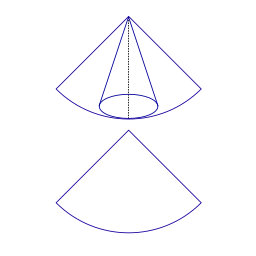All cones have a radius, slant height, and height.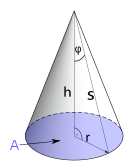The illustration above shows a cone with a radius, slant height, and height. The radius of the cone is the radius of the circle located at the bottom. The slant height is the distance alone the side of the cone from the bottom to the top, while the height is the distance from the center of the circle to the bottom of the cone.

Now picture that same shape that was spread out from a cone and imagine the tip of it being cut off, then wrap the sides to touch again as they were before when it formed the cone. This resulting shape is called a truncated cone. It is a special type of cone that has two circles: one on the top and one on the bottom. Similar to regular cones, truncated cones have a radius, slant height, and height. However, truncated cones have two radii, one for the top circle and another for the bottom.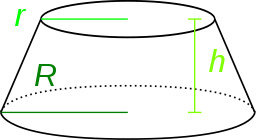Truncated cones are the shapes used for funnels, where it has a cone shape but an opening on the top and on the bottom to let matter flow through it. However, these do not necessarily have to be 'openings' for it to be considered a truncated cone. Picture a popcorn container for example. It has an opening on the top, but the bottom is closed to keep the popcorn contained. This shape is still considered a truncated cone.

## Truncated Cone Definition

What was the last really great film you went to see at a movie theater? Going to the movies is always a great time! Of course, the experience of going to a movie at the theater isn't complete without popcorn! Most likely, you've never taken note of the shape of a popcorn tub at the theater, but here's an interesting fact! There is a mathematical name for this shape, and it's called a truncated cone.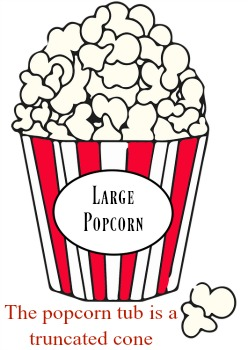A truncated cone is a three-dimensional object that looks like a cone with the top pointy end chopped off.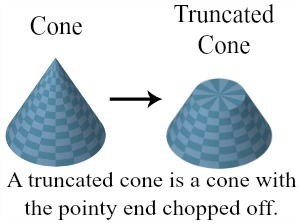The two circular ends of a truncated cone are called the bases. We will call the radius of the smaller circular base the little radius and represent it with r, and we will call the radius of the larger circular base the big radius and represent it with R. The distance between the centers of the two bases is called the height of the truncated cone, and we represent it with h. Lastly, the shortest distance between the outer edges of the bases is called the slant height, and we represent it with s.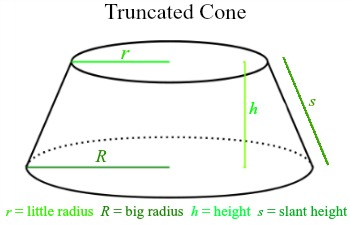Do you now see that a popcorn tub has this shape? Pretty interesting, huh? Well, it's about to get even more interesting! Let's talk a bit about the volume and surface area of a truncated cone!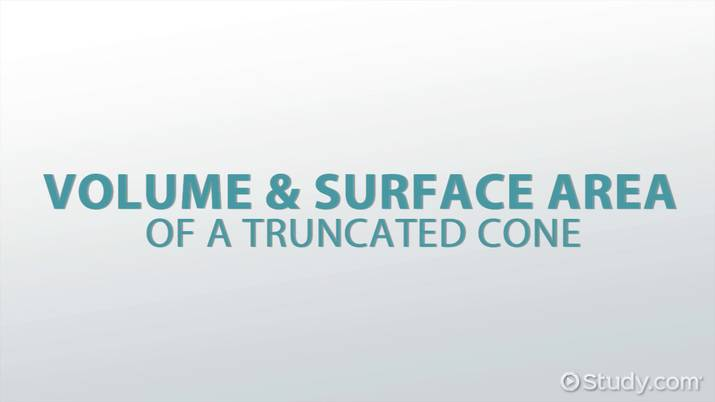An error occurred trying to load this video.

Try refreshing the page, or contact customer support.

Coming up next: Surface Area of a Triangular Prism

### You're on a roll. Keep up the good work!

Replay
Your next lesson will play in 10 seconds
• 0:04 Truncated Cone Definition
• 1:12 Truncated Cone Volume
• 2:42 Truncated Cone Surface Area
• 5:03 Lesson Summary
Save Save

Want to watch this again later?

Timeline
Autoplay
Autoplay
Speed Speed

## How to Find the Area of a Cone?

Recall that area describes the amount of space contained in a two-dimensional shape, and it is always expressed in units squared. Calculating an area usually involves multiplying the length of the shape by the height. For triangles, the area is found by multiplying the base by the height of the triangle.

For three dimensional shapes, there are two types of areas: Lateral surface area and total surface area. The lateral surface area of a shape is the area around the sides of the shape. For a cone, the lateral surface area would be the area of the fan shape that wraps around to form the cone. Lateral surface area can be thought of as the surface area without the area of the base(s).

What about the area of the base? What if that were to be included? That is where total surface area comes in. The total surface area of a shape includes both the lateral surface area as well as the area of the base(s). It is, as the name suggests, the total surface area of the entire shape and includes every side of the shape. Total surface area can be found by adding the lateral surface area to the area of the base(s).

## Surface Area of a Cone Formula

As previously mentioned, the lateral surface area for a cone is found by finding the area of the fan-like shape that forms the cone. For the case of a cone, the height of the fan shape would be the slant height of the cone, while the base of the fan shape comes from the circle that is formed at the base of the cone. The formula for the lateral area of a cone is the following:

{eq}\text{A}_{l} = \pi r s {/eq}

where {eq}\text{A}_{l} {/eq} is the lateral surface area of a cone, {eq}r {/eq} is the circle radius of the cone while {eq}s {/eq} is the slant height of the cone.

If the slant height of a cone is unknown but the radius and height of the cone are provided, then the slant height can be solved for using the following formula:

{eq}s = \sqrt{ r^2 + h^2 } {/eq}

where {eq}r {/eq} is the circle radius of a cone while {eq}h {/eq} is the height of a cone.

Using this formula for the slant height of the cone and plugging it in for the lateral area, the result is the following formula:

{eq}\text{A}_{l} = \pi r \sqrt{r^2 + h^2} {/eq}

Using this formula, the lateral surface area of a cone can be solved for if the slant height is not provided.

The total surface area of a cone can be found by adding the lateral surface area to the base surface area of the cone. The area of a circle can be found from the following formula:

{eq}\text{Circle area} = \pi r^2 {/eq}

where {eq}r {/eq} is the radius of the circle. Adding this formula to the lateral surface area of a cone formula yields the total surface area of a cone:

{eq}\text{A}_{t} = \text{S}_{l} + \pi r^2 {/eq}

or

{eq}\text{A}_{t} = \pi r \sqrt{r^2 + h^2} + \pi r^2 {/eq}

Using these formulas, one could solve for the lateral and total surface area of a cone.

### How to Calculate the Surface Area of a Cone?

When calculating the surface area of a cone, there are specifics that must be taken into consideration. Take the following example:

Example
A cone has a radius of {eq}4\text{in} {/eq} and a height of {eq}7\text{in} {/eq}, what is the lateral and total surface area of this cone?

→ Solution:

The lateral surface area can be found by plugging in the values for radius and height into the formula for lateral surface area of a cone:

{eq}\text{A}_{l}= \pi r \sqrt{ r^2 + h^2 } \\ \text{A}_{l}= \pi 4 \sqrt{16 + 49} \\ \text{A}_{l}= 12.57\sqrt{65} \\ \text{A}_{l} \approx 101.34\text{in}^{2} \\ {/eq}

Now that the lateral surface area was solved for, this value can be used to calculate the total surface area of the cone:

{eq}\text{A}_{t} = \text{A}_{l} + \pi r^2 \\ \text{A}_{t} = 101.34 + \pi r^2 \\ \text{A}_{t} = 101.34 + \pi 4^2 \\ \text{A}_{t} = 101.34 + 16\pi \\ \text{A}_{t} \approx 151.61\text{in}^{2} {/eq}

Therefore, the lateral surface area of the cone is about {eq}101.34 \text{in}^2 {/eq} while the total surface area of the cone is about {eq}151.61 \text{in}^2 {/eq}

## Surface Area of Truncated Cone

The surface areas for truncated cones are slightly different than the surface areas of regular cones due to the differences within the shapes. To find the lateral surface area of a truncated cone, the following formula can be used:

{eq}\text{A}_{l\text{ }t} = \pi (R + r) s {/eq}

where {eq}\text{A}_{l\text{ }t} {/eq} is the lateral surface area for a truncated cone, {eq}R {/eq} is the radius of the larger circle, {eq}r {/eq} is the radius of the smaller circle, and {eq}s {/eq} is the slant height of the truncated cone. Similar to the slant height of a regular cone, the truncated cone slant height can be calculated using the values for the height and radii of the truncated cone:

{eq}s = \sqrt{h^2 + (R - r )^2} {/eq}

where {eq}s {/eq} is the slant height of a truncated cone and {eq}h {/eq} is the height of the truncated cone.

To find the total surface area of a truncated cone, simply add the lateral surface area to the areas of both bases. Unlike regular cones, truncated cones have two bases since they have two circles. The total surface area formula for truncated cones is the following:

{eq}\text{A}_{t\text{ }t} = \pi [ s (R + r) + R^2 + r^2] {/eq}

where {eq}\text{A}_{t\text{ }t} {/eq} is the total surface area of a truncated cone. Plugging in the formula for {eq}s {/eq}, the formula for the total surface area of a truncated cone can also be written as the following:

{eq}\text{A}_{t\text{ }t} = \pi \sqrt{h^2 + (R - r )^2} \text{ }(R + r) + R^2 + r^2 {/eq}

Using these formulas, the total surface area or the lateral surface area of a truncated cone can be solved for.

### How to Calculate the Surface Area of a Truncated Cone?

Calculating the surface area of truncated cone is a little different. Take the following example:

Example
A truncated cone has a larger radius of {eq}5\text{in} {/eq} and a smaller radius of {eq}3\text{in} {/eq}. The height of the truncated cone is {eq}10\text{in} {/eq}. What is the lateral and total surface area of this truncated cone?

→ Solution:

## Truncated Cone Volume

Suppose you go to the movies, and you get a large popcorn. You can't wait to sink your teeth into the salty, buttery goodness, but before doing so, you begin to wonder just how much popcorn fits inside the large popcorn tub. I've got news for you! You're actually wondering what the volume of the popcorn tub is!

The volume of a truncated cone is the amount of space inside the truncated cone. The good news is that we have a nice formula that we can use to find the volume of a truncated cone.

Volume = (1/3) ⋅ π ⋅ h(R 2 + r 2 + Rr)

Your curiosity gets the best of you, so you ask the worker at the concessions counter to see an empty large popcorn tub. When he gives it to you, you take some quick measurements and find that the big radius is 5 inches, the small radius is 4 inches, the height is 10 inches, and the slant height is 11 inches. You give the empty tub back to the concessions worker and pull out a pencil and some paper to calculate the volume of the large popcorn tub using your formula.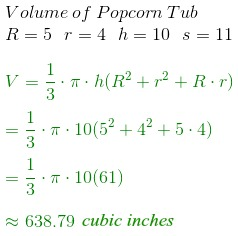So, we see that the volume of the large popcorn tub is approximately 638.79 cubic inches. Wow! That's a lot of popcorn! Dig in!

As you begin to gobble down your popcorn, you begin to have a bit of buyer's remorse. After all, you paid $8 for this large popcorn, and that's not cheap! This gets you to wondering just how much the movie theater has to pay for a popcorn tub. You have a bit more time until your movie starts, so you ask a worker how much these tubs cost them. The worker says that the manufacturer charges based on how much material was used to make the tub and a lid for the tub, and they charge 0.00125 cents per square inch of material used. To unlock this lesson you must be a Study.com Member. Create your account Video Transcript ## Truncated Cone Definition What was the last really great film you went to see at a movie theater? Going to the movies is always a great time! Of course, the experience of going to a movie at the theater isn't complete without popcorn! Most likely, you've never taken note of the shape of a popcorn tub at the theater, but here's an interesting fact! There is a mathematical name for this shape, and it's called a truncated cone.A truncated cone is a three-dimensional object that looks like a cone with the top pointy end chopped off.The two circular ends of a truncated cone are called the bases. We will call the radius of the smaller circular base the little radius and represent it with r, and we will call the radius of the larger circular base the big radius and represent it with R. The distance between the centers of the two bases is called the height of the truncated cone, and we represent it with h. Lastly, the shortest distance between the outer edges of the bases is called the slant height, and we represent it with s.Do you now see that a popcorn tub has this shape? Pretty interesting, huh? Well, it's about to get even more interesting! Let's talk a bit about the volume and surface area of a truncated cone! ## Truncated Cone Volume Suppose you go to the movies, and you get a large popcorn. You can't wait to sink your teeth into the salty, buttery goodness, but before doing so, you begin to wonder just how much popcorn fits inside the large popcorn tub. I've got news for you! You're actually wondering what the volume of the popcorn tub is! The volume of a truncated cone is the amount of space inside the truncated cone. The good news is that we have a nice formula that we can use to find the volume of a truncated cone. Volume = (1/3) ⋅ π ⋅ h(R 2 + r 2 + Rr) Your curiosity gets the best of you, so you ask the worker at the concessions counter to see an empty large popcorn tub. When he gives it to you, you take some quick measurements and find that the big radius is 5 inches, the small radius is 4 inches, the height is 10 inches, and the slant height is 11 inches. You give the empty tub back to the concessions worker and pull out a pencil and some paper to calculate the volume of the large popcorn tub using your formula.So, we see that the volume of the large popcorn tub is approximately 638.79 cubic inches. Wow! That's a lot of popcorn! Dig in! ## Truncated Cone Surface Area As you begin to gobble down your popcorn, you begin to have a bit of buyer's remorse. After all, you paid$8 for this large popcorn, and that's not cheap! This gets you to wondering just how much the movie theater has to pay for a popcorn tub. You have a bit more time until your movie starts, so you ask a worker how much these tubs cost them. The worker says that the manufacturer charges based on how much material was used to make the tub and a lid for the tub, and they charge 0.00125 cents per square inch of material used.

To unlock this lesson you must be a Study.com Member.

#### What are the properties of a truncated cone?

Truncated cones are similar to regular cones, except they have two bases rather than one. They have a larger circle on one end and a smaller circle on the other.

#### What is the area and volume of a cone?

The area of a cone is A = πr√(r² + h²) + πr² while the volume of a cone is V = πr² ⋅ h⁄3. Area is always expressed in units squared while volume is expressed in units cubed.

### Register to view this lesson

Are you a student or a teacher?

Back

### Resources created by teachers for teachers

Over 30,000 video lessons & teaching resources‐all in one place.Video lessonsQuizzes & WorksheetsClassroom IntegrationLesson Plans

I would definitely recommend Study.com to my colleagues. It’s like a teacher waved a magic wand and did the work for me. I feel like it’s a lifeline.

Jennifer B.
TeacherCreate an account to start this course today
Used by over 30 million students worldwide